Ben Lee

•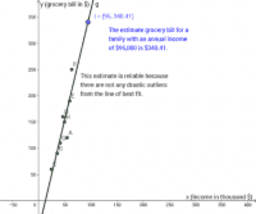Midterm II Question 2 (Ben Lee)

Activity

Ben Lee

•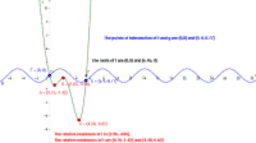Midterm II Question 1 (Ben Lee)

Activity

Ben Lee

•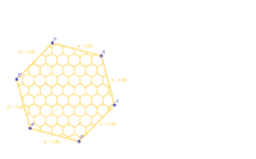Geogebra Assignment #8 Q4 (Ben Lee)

Activity

Ben Lee

•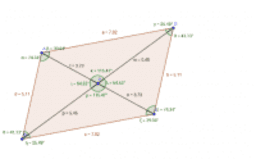Geogebra Assignment #8 Q3 (Ben Lee)

Activity

Ben Lee

•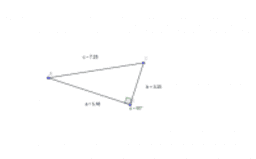Geogebra Assignment #8 Q2 (Ben Lee)

Activity

Ben Lee

•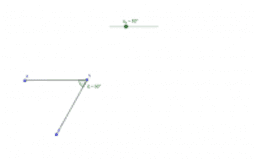Geogebra Assignment #8 Q1 (Ben Lee)

Activity

Ben Lee

•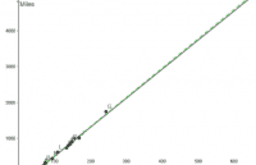Geogebra Assignment #5 Q2

Activity

Ben Lee

•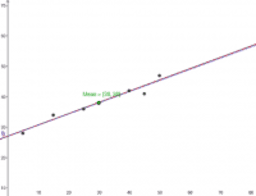Geogebra Assignment #5 Q1 (Ben Lee)

Activity

Ben Lee

•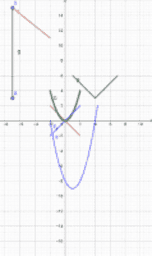Geogebra Assignment #2 (Ben Lee)

Activity

Ben Lee

•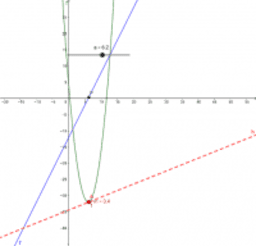Geogebra Assignment #4 Q2 (Ben Lee)

Activity

Ben Lee

•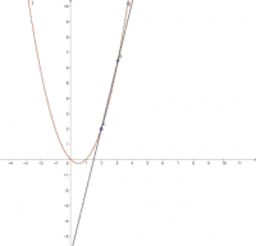Geogebra Assignment #4 Q1 (Ben Lee)

Activity

Ben Lee

•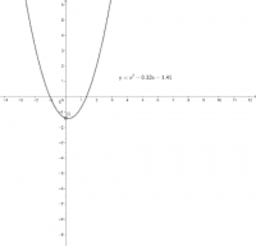Geogebra Assignment #3 Q3 (Ben Lee)

Activity

Ben Lee

•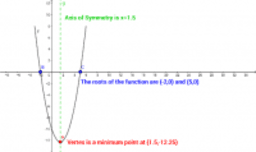Geogebra Assignment #3 Q1&2 (Ben Lee)

Activity

Ben Lee

•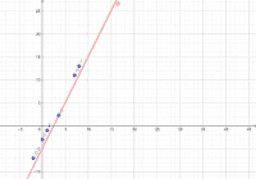Geogebra Assignment #1 (Ben Lee)

Activity

Ben Lee

•Geogebra Assignment #1 (Ben Lee)

Activity

Ben Lee

•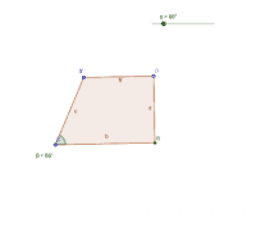Midterm II Question 4 (Ben Lee)

Activity

Ben Lee

•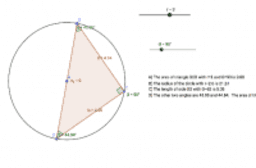Activity

Ben Lee